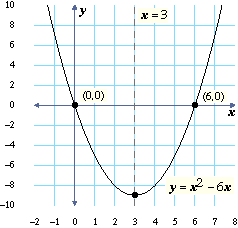# Investigating \$y=ax^2+bx\$

In this lesson, we’ll go back to studying graphs of quadratic equations. You will learn about the shapes of the graphs of equations such as \$y=x^2+x\$, and how they relate to other properties of those equations.

## Roots and axis of symmetry

Remember that the roots or zeros of an equation are the \$x\$-coordinates of the points where its graph touches the \$x\$-axis.

 What are the roots of the equation you are looking at to the left, \$y=x^2-6x\$? (Notice that the graph is zoomed out somewhat: its grid lines mark even integers between \$-10\$ and \$10\$, instead of all integers between \$-5\$ and \$5\$ as usual.)

Also remember that the axis of symmetry of a parabola is the line that you could fold the parabola along so that its two sides would match each other.

 What is the axis of symmetry of \$y=x^2-6x\$?

Use the sliders to change the values of \$a\$ and \$b\$ in the equation \$y=ax^2+bx\$ and answer the following questions.

 What are the shapes of the red graphs you are looking at?

Complete the following table.

Equation\$a\$\$b\$rootsaxis of
symmetry
 Look at the table you completed for quadExs and at the sliders and graph to the left. The axis of symmetry of a parabola is between its two roots (\$x\$-intercepts). Is it closer to the left root, closer to the right root, or right in the middle?
 If the roots of a parabola are at 0 and 12, where is its axis of symmetry?
 Where is the axis of symmetry of a parabola with roots at 0 and \$-12\$?

You can also find the roots of an equation without graphing, as in the following example. To find the roots and axis of symmetry of \$y=x^2-3x\$ without graphing, use factoring (or the distributive law), as follows.

The roots are where \$y=0\$, so we have
\$x^2-3x=0\$ which factors into:
\$x(x-3)=0\$. So, \$x=0\$ OR \$x-3=0\$ → \$x=3\$

Roots: The roots of \$y=x^2-3x\$ are 0 and 3.

Axis of symmetry: The axis of symmetry of a parabola passes midway between its roots, so the axis of symmetry of this parabola is the line \$x=1.5\$.

 Find the roots of \$y=x^2-4x\$ by factoring.
 Use these roots to find the axis of symmetry of \$y=x^2-4x\$.

Use the sliders and graph to the left to check your answers.

In the next few questions, we will find the roots of the general equation \$y=ax^2+bx\$ with \$a≠0\$ by factoring, and use that to get a formula for the axis of symmetry of any equation in that form.

 We want to factor \$ax^2+bx\$. Because both terms contain an \$x\$, one of the factors will be \$x\$. What is the other factor?
 Since one of the factors is \$x\$, one of the roots of the equation is \$x=0\$. The other factor is \$ax+b\$. What is the other root of the equation? (That is, what \$x\$-value solves \$ax+b=0\$?)
 The axis of symmetry is halfway between the two roots (0 and \$\$-b/a\$\$). Complete the equation to the right for the axis of symmetry. (Hint: For any number \$n\$, the number halfway between \$0\$ and \$n\$ is \$\$n/2\$\$.)

Your results from quadExs have been copied into the table below. Complete this table.

Equation\$a\$\$b\$axis of
symmetry
\$\$-b/{2a}\$\$

As you can see:

The axis of symmetry of \$y=ax^2+bx\$ is the line \$\$x=-b/{2a}\$\$.

Below you can see the graph of \$y=x^2-6x\$. The axis of symmetry of this parabola is the line:

\$\$x = {-b}/{2a} = {-(-6)}/{2(1)} = 6/2 = 3\$\$We want to find the vertex of this parabola. The vertex is on the axis of symmetry, so its \$x\$-coordinate is 3. The vertex is also a point on the parabola, so it satisfies the equation for the parabola. This means that if you plug the \$x\$-coordinate of the vertex into the equation, you will get the \$y\$-coordinate.

Plugging 3 for \$x\$ into \$y=x^2-6x\$ gives \$y=(3)^2-6(3)\$ → \$y=9-18\$ → \$y=-9\$
So the vertex is at the point \$(3,-9)\$.

 Find the roots of \$y=2x^2-4x\$ by factoring.
 What is the axis of symmetry of this parabola?
 Use the method described above to find the vertex of \$y=2x^2-4x\$.
 Now, we’ll look at the equation \$y=-2x^2+8x\$. Find the roots of this equation by factoring.
 What is the axis of symmetry of this parabola?
 What is the vertex of this parabola?

You can check your answers using the sliders and graph to the left.